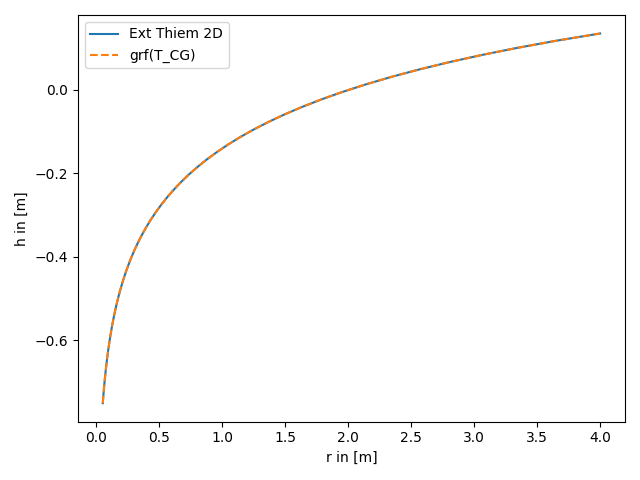# extended Thiem 2D vs. steady solution for coarse graining transmissivity

The extended Thiem 2D solutions is the analytical solution of the groundwater flow equation for the coarse graining transmissivity for pumping tests. Therefore the results should coincide.

References:```import numpy as np
from matplotlib import pyplot as plt

from anaflow.tools.coarse_graining import T_CG

rad = np.geomspace(0.05, 4)  # radius from the pumping well in [0, 4]
r_ref = 2.0  # reference radius

var = 0.5  # variance of the log-transmissivity
len_scale = 10.0  # correlation length of the log-transmissivity
TG = 1e-4  # the geometric mean of the transmissivity

rate = -1e-4  # pumping rate

rad, r_ref, T_CG, rate=rate, trans_gmean=TG, var=var, len_scale=len_scale
)